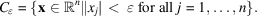International
Tables for
Crystallography
Volume B
Reciprocal space
Edited by U. Shmueli

International Tables for Crystallography (2010). Vol. B, ch. 1.3, p. 42   | 1 | 2 |

## Section 1.3.2.6.1. Terminology

G. Bricognea

aGlobal Phasing Ltd, Sheraton House, Suites 14–16, Castle Park, Cambridge CB3 0AX, England, and LURE, Bâtiment 209D, Université Paris-Sud, 91405 Orsay, France

#### 1.3.2.6.1. Terminology

| top | pdf |

Letbe the subset ofconsisting of those points with (signed) integer coordinates; it is an n-dimensional lattice, i.e. a free Abelian group on n generators. A particularly simple set of n generators is given by the standard basis of, and hencewill be called the standard lattice in. Any other nonstandard' n-dimensional lattice Λ inis the image of this standard lattice by a general linear transformation.

If we identify any two points inwhose coordinates are congruent modulo, i.e. differ by a vector in, we obtain the standard n-torus. The latter may be viewed as, i.e. as the Cartesian product of n circles. The same identification may be carried out modulo a nonstandard lattice Λ, yielding a nonstandard n-torus. The correspondence to crystallographic terminology is that standard' coordinates over the standard 3-torusare called `fractional' coordinates over the unit cell; while Cartesian coordinates, e.g. in ångströms, constitute a set of nonstandard coordinates.

Finally, we will denote by I the unit cubeand bythe subset